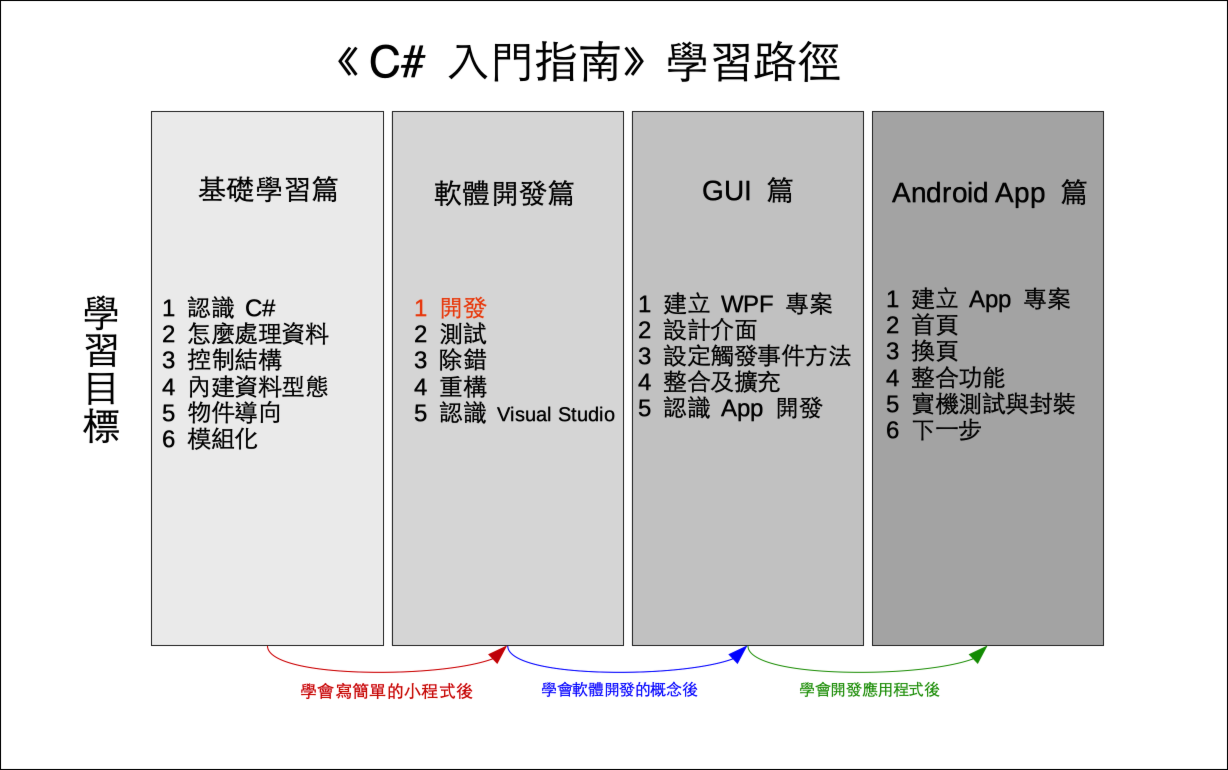# 單元 19 - 解碼

～～學習進度表～～Tbana kw dm wvuur.
×

 0 1 2 3 4 5 6 7 8 9 10 11 12 13 14 15 16 17 18 19 20 21 22 23 24 25 a b c d e f g h i j k l m n o p q r s t w u v x y z

``````// 解碼方法
public string ToDecode(string s)
{
// 建立暫存字串
string result = "";

// 第一層迴圈逐一取得字元
for (int i = 0; i < s.Length; i++)
{
// 判斷是否為英文小寫字母
if (s[i] >= 97 && s[i] <= 122)
{
// 第二層迴圈進行解碼轉換
for (int j = 0; j < 26; j++)
{
// 找到字元在密碼表中的索引值
if (s[i] == code[j])
{
result += Convert.ToChar(j + 97);
break;
}
}
}
// 不是英文小寫字母，直接將字元附加到 result 之後
else
{
result += s[i];
}
}

// 回傳結果
return result;
}``````

ToDecode()ToEncode() 相似，同樣需要一個字串 (string) 當參數 (parameter) ，結果也回傳一個字串。

``````// 第一層迴圈逐一取得字元
for (int i = 0; i < s.Length; i++)
{
// 判斷是否為英文小寫字母
if (s[i] >= 97 && s[i] <= 122)
{
// 第二層迴圈進行解碼轉換
for (int j = 0; j < 26; j++)
{
// 找到字元在密碼表中的索引值
if (s[i] == code[j])
{
result += Convert.ToChar(j + 97);
break;
}
}
}
// 不是英文小寫字母，直接將字元附加到 result 之後
else
{
result += s[i];
}
}``````

Encrypt 類別 (class) 到這邊大致已開發完成，完整程式如下

``````using System;

namespace EncryptDemo07
{
class EncryptDemo07
{
// 宣告密碼表欄位
public string code;

// 建構子，呼叫建立密碼表的 SetCode() 方法
public EncryptDemo07()
{
SetCode();
}

// 建立密碼表
public void SetCode()
{
// 宣告變數
int a, b, x, y, m;
char c = 'a';
// a 、 b 初始化
Random r = new Random();
a = 0;
b = 0;
// a 不能是 0 或偶數
while (a % 2 == 0)
{
a = r.Next(1, 10);
b = r.Next(0, 10);
}
code = "";
// 建立密碼表的迴圈
for (int i = 0; i < 26; i++)
{
x = c;
y = x * a + b;
m = y % 26;
code += Convert.ToChar(m + 97);
c++;
}
}

// 編碼方法
public string ToEncode(string s)
{
// 建立暫存字串
string result = "";

char c;
int m;
// 逐一取得字元進行處理
for (int i = 0; i < s.Length; i++)
{
// 判斷是否為英文小寫字母
if (s[i] >= 97 && s[i] <= 122)
{
c = s[i];
m = c - 97;
result += code[m];
}
// 不是英文小寫字母，直接將字元附加到 result 之後
else
{
result += s[i];
}
}

// 回傳結果
return result;
}

// 解碼方法
public string ToDecode(string s)
{
// 建立暫存字串
string result = "";

// 第一層迴圈逐一取得字元
for (int i = 0; i < s.Length; i++)
{
// 判斷是否為英文小寫字母
if (s[i] >= 97 && s[i] <= 122)
{
// 第二層迴圈進行解碼轉換
for (int j = 0; j < 26; j++)
{
// 找到字元在密碼表中的索引值
if (s[i] == code[j])
{
result += Convert.ToChar(j + 97);
break;
}
}
}
// 不是英文小寫字母，直接將字元附加到 result 之後
else
{
result += s[i];
}
}

// 回傳結果
return result;
}
}

class Program
{
static void Main(string[] args)
{
// 建立密碼物件
EncryptDemo07 e = new EncryptDemo07();
// 印出密碼表
Console.WriteLine(e.code);
// 建立測試字串
string s1 = "There is no spoon.";
// 印出測試字串
Console.WriteLine(s1);
// 進行編碼
string s2 = e.ToEncode(s1);
// 印出編碼結果
Console.WriteLine(s2);
// 進行解碼
string s3 = e.ToDecode(s2);
// 印出解碼結果
Console.WriteLine(s3);
}
}
}

//《程式語言教學誌》的範例程式
// http://kaiching.org/
// 專案：EncryptDemo07
// 檔名：Program.cs
// 功能：示範利用 C# 設計 Encrypt 類別
// 作者：張凱慶``````

``````// 進行解碼
string s3 = e.ToDecode(s2);
// 印出解碼結果
Console.WriteLine(s3);``````

 C:\EncryptDemo07> dotnet run venwfoxgpyhqzirajsbktcludm There is no spoon. Tgfsf pb ir barri. There is no spoon. C:\EncryptDemo07>1. C# 程式設計手冊 | Microsoft Docs - 類別

1. 解碼與編碼用到相同的轉換表格，因為索引值 0 等於 'a' ，索引值 1 等於 'b' ，餘下可類推。
2. 巢狀迴圈是指迴圈中有其他迴圈，因為用縮排的方式編排程式碼，凹陷下去的地方看起來像鳥巢，故有此名。
3. 解碼利用巢狀迴圈進行，第一層迴圈逐一取得每一個字元，第二層迴圈尋找該字元在密碼表中的索引值。

1. 為什麼編碼跟解碼可以用一樣的轉換表格？
2. 可以不用巢狀迴圈來解碼嗎？
3. 巢狀迴圈可以用一樣名稱的控制變數嗎？

1. 承接上一個單元的猜數字遊戲，將新專案寫在 Exercise1901 中，把答案 answerShuffle() 攪亂順序，然後用 Substring(0, 4) 取前四個數字當答案。
2. 承上題，將新專案寫在 Exercise1901 中，修正第一位數可能為 0 的情況。